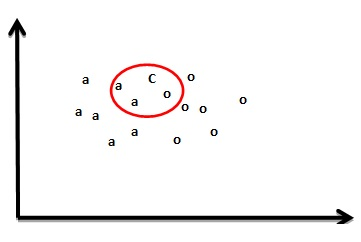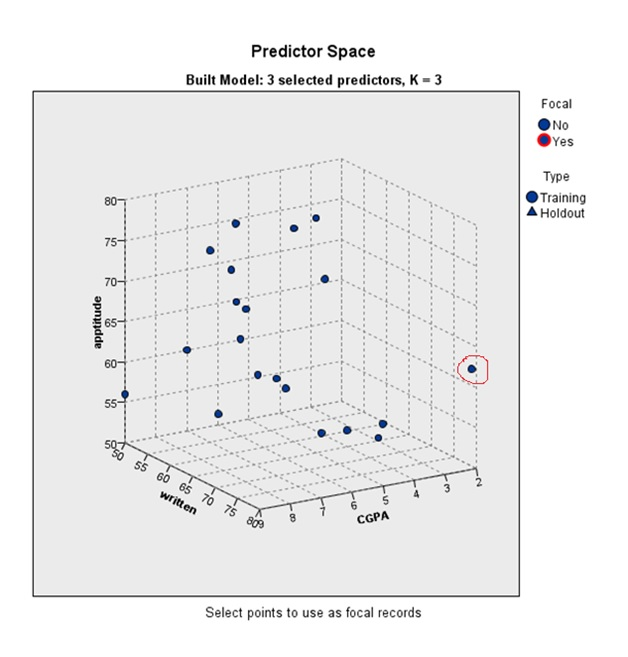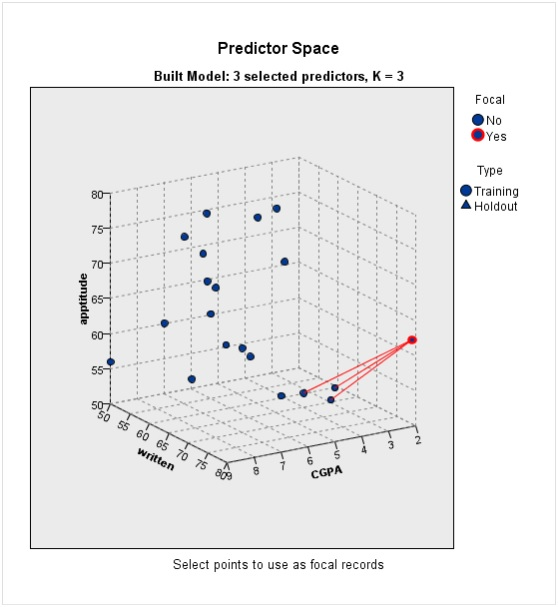How to use K-Nearest Neighbor (KNN) algorithm on a dataset?

K- Nearest Neighbor, popular as K-Nearest Neighbor (KNN), is an algorithm that helps to assess the properties of a new variable with the help of the properties of existing variables. KNN is applicable in classification as well as regression predictive problems. KNN is a simple non-parametric test. It does not involve any internal modeling and does not require data points to have certain properties. It simply takes the voting of majority of variables and accordingly treats new variables.

For example, a company manufactures tissue papers and tests it for acid durability and strength. Depending upon the test results, it classifies the new paper tissues either ‘good’ or ‘bad’. Now, if the company produces a type of tissue paper it can use K-Nearest Neighbor to decide the labels for newly produced tissues.

How K-Nearest Neighbor (KNN) works?

Suppose there is a scatter plot of two variables, ‘a’ and ‘o’. Then a third variable, ‘c’ is introduced to the scatter plot. Now to label this variable as existing ones, KNN can be applied.Figure 1: Scatter plot of variables for K-Nearest Neighbor (KNN) example

To start with KNN, consider a hypothesis of the value of ‘K’. Suppose K = 3 in this example. Here, K is the nearest neighbor and wishes to take vote from three existing variables. Therefore, K Nearest Neighbor will be used. The variable ‘c’ will be encircled taking three more existing variables which are nearest.Figure 2 : Scatterplot of variables in KNN example

For instance, using KNN with K = 3, the three nearest neighbors of new variable ‘c’ were encircled. Then, a vote from the three selected nearest neighbors show that there are two ‘a’ and one ‘o’ variable. Since variable ‘a’ is more in number than variable ‘o’, the new variable ‘c’ must be labeled as ‘a’.

Numerical example of KNN in SPSS

This section gives an example to show the application of K-Nearest Neighbor algorithm in SPSS. The chosen dataset contains various test scores of 30 students. So, on the basis of these scores, K Nearest Neighbor test can be used to find the nearest neighbor for ‘application status’. In this case the variable ‘status’ has only two values; 1- hired and 0- not hired.Table 1 Data set used for KNN test in SPSS

As discussed above, the KNN test uses the nearest value to predict the target variable. For example the value of the point shown in the figure below can be predicted (marked in red).Figure 3: Predicted value of point (in red)Figure 4: Nearest values from the point (in red)

The result above shows three red lines leading to the three nearest values from the point. The X-axis (written score), Y-axis (aptitude score) and Z-axis (CGPA) are the variables for prediction.Figure 5: Peer chart for KNN test in SPSS

Similarly the peer chart shows which value is used from which variable to predict the new variable based on the nearest value. In the peer chart the values in red are the nearest values for predicting the new variable whereas the blue dots values are idle.  The numbering within the chart represents the respondent. For example 1 is the data for the first respondent, which the algorithm uses to predict values or groups in the response variable. Peer chart also shows the data which is to be used for training the model and left for validation. So far there is no Holdout data in this dataset and all the data is used for training the KNN model.

Thus, K Nearest Neighbor helped in classifying the applicants in two groups (i.e. hired, not hired) based on their acquired CGPA, aptitude and written tests. It helped the hiring company to easily collect the data containing candidate’s information and evaluate it accordingly.

Applications of K-Nearest neighbor

Apart from using as classification tool like described above, KNN has several further applications in practical world:

• It is popular in search applications. For example, if one wants to find the most similar documents to a certain document, i.e. for detecting plagiarism, KNN algorithm is ideal.
• It is also applicable in recommender systems in order to search for items which are similar to those in demand by other users. Here, K Nearest Neighbor will help deduce that items liked commonly by two or more people tend to be similar.
• The algorithm also has many uses in data mining and machine learning. One particular use of K Nearest Neighbor is in anomaly detection. Anomaly detection is the identification of items, events, or observations which do not conform to the expected patterns or other items in a dataset.

Software that support K-Nearest Neighbor best are R, SAS, MATLAB, STATA and SPSS.

Prateek Sharma

Analyst at Project Guru
Prateek has completed his graduation in commerce with a rich experience in Telecom, Marketing and Banking domains for preparing comprehensive documents and reports while managing internal and external data analysis. He is an adaptable business-minded Data Analyst at Project Guru skilled in recording, interpreting and analysing data with a demonstrated ability to deliver valuable insights via data analytics and advanced data-driven methods. Apart from his strong passion towards data science, he finds extreme sports interesting. He keeps himself updated with the latest tech and always love to learn more about latest gadgets and technology.

Related articles

• How to apply linear discriminant analysis? Linear discriminant model is a multivariate model. It is used for modeling the differences in groups. In this model a categorical variable can be predicted through continuous or binary dependent variable.
• Understanding random operating curves or ROC analysis Receiver Operating Curve (ROC) is an extension of such classifications. Performance of binary classifier system in the case of ROC analysis can be tested.
• Solving complicated problems with decision tree A decision tree is a graphical representation of possible solutions to a problem based on given conditions. It is called a tree because diagrammatically it starts with a single box (target variable) and ends up in numerous branches and roots (numerous solutions).
• How to conduct generalized least squares test? In statistics, Generalized Least Squares (GLS) is one of the most popular methods for estimating unknown coefficients of a linear regression model when the independent variable is correlating with the residuals.
• How to perform LASSO regression test? In statistics, to increase the prediction accuracy and interpret-ability of the model, LASSO (Least Absolute Shrinkage and Selection Operator) is extremely popular. It is a regression procedure that involves selection and regularisation and was developed in 1989. Lasso regression is an […]We are looking for candidates who have completed their master's degree or Ph.D. Click here to know more about our vacancies.